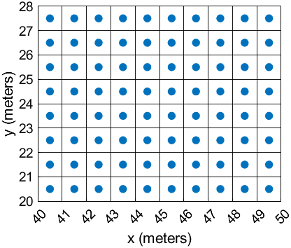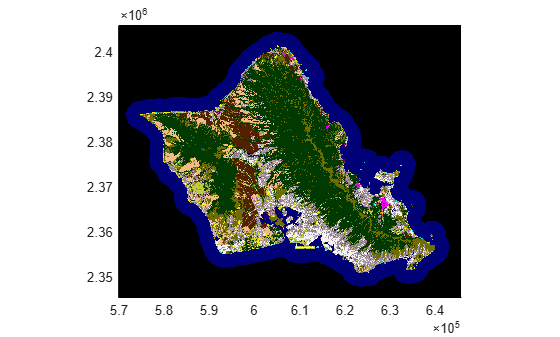# MapCellsReference

Reference raster cells to map coordinates

## Description

A map cells reference object contains information that describes the relationship between a planar map coordinate system and an intrinsic coordinate system that is anchored to the columns and rows of a 2-D spatially referenced raster grid or image of cells.

Typically, the raster is sampled regularly in the planar world x and world y coordinates of the map system, such that the intrinsic x and world x axes align and the intrinsic y and world y axes align. When this is true, the relationship between the two systems is rectilinear. More generally, and much more rarely, their relationship is affine. The affine relationship allows for a possible rotation (and skew). In either case, rectilinear or affine, the sample spacing from row to row need not equal the sample spacing from column to column. The cells or pixels need not be square. In the most general case, they could conceivably be parallelograms, but in practice they are always rectangular.

This image illustrates a 8-by-10 planar raster of cells. The raster cells have an extent of 1 meter in x and y, cover x values in the range [40,50] in meters, and cover y values in the range [20,28] in meters. The boundary of the raster of cells is made up of the outermost boundaries of the outermost cells.To reference a raster of postings to planar map coordinates, use a `MapPostingsReference` object instead.

## Creation

You can use these functions to create `MapCellsReference` objects:

## Properties

expand all

Limits of raster in world x-coordinates, specified as a two-element row vector of the form `[xMin xMax]`.

The value of the `ProjectedCRS` property determines the length units for the raster. This code shows how to find the length units for a raster associated with the map cells reference object `R`.

`R.ProjectedCRS.LengthUnit`

Example: `[207000 209000]`

Data Types: `double`

Limits of raster in world y-coordinates, specified as a two-element row vector of the form `[yMin yMax]`.

The value of the `ProjectedCRS` property determines the length units for the raster. This code shows how to find the length units for a raster associated with the map cells reference object `R`.

`R.ProjectedCRS.LengthUnit`

Example: `[911000 913000]`

Data Types: `double`

Number of rows and columns of the raster or image associated with the referencing object, specified as a two-element vector, [m n], where m represents the number of rows and n the number of columns.

For convenience, you can assign a size vector having more than two elements. This enables assignments like ```R.RasterSize = size(RGB)```, where `RGB` is m-by-n-by-3. In cases like this, the object stores only the first two elements of the size vector and ignores the higher (nonspatial) dimensions.

Example: `[200 300]`

Data Types: `double`

Geometric nature of the raster, specified as `'cells'`. The value `'cells'` indicates that the raster comprises a grid of quadrangular cells, and is bounded on all sides by cell edges. For an m-by-n raster, points with an intrinsic x-coordinate of 1 or n or an intrinsic x-coordinate of 1 or m fall within the raster, not on its edges.

Data Types: `char`

Edge from which column indexing starts, specified as `'south'` or `'north'`.

Example: `'south'`

Data Types: `char`

Edge from which row indexing starts, specified as `'west'` or `'east'`.

Example: `'east'`

Data Types: `char`

Extent in world x-coordinates of individual cells, specified as a positive numeric scalar. Distance between the eastern and western limits of a single raster cell. The value is the same for all cells in the raster.

Example: `2.5`

Data Types: `double`

Extent in world y-coordinates of individual cells, specified as a positive numeric scalar. Distance between the northern and southern limits of a single raster cell. The value is the same for all cells in the raster.

Example: `2.5`

Data Types: `double`

Extent of the full raster or image as measured in the world system in a direction parallel to its rows, specified as a positive numeric scalar. In the case of a rectilinear geometry, which is most typical, this is the horizontal direction (east-west).

Data Types: `double`

Extent of the full raster or image as measured in the world system in a direction parallel to its columns, specified as a positive numeric scalar. In the case of a rectilinear geometry, which is most typical, this is the vertical direction (north-south).

Data Types: `double`

Raster limits in intrinsic x-coordinates, specified as a two-element row vector of positive integers, ```[xMin xMax]```. For an m-by-n raster, `XIntrinsicLimits` equals ```[0.5, m+0.5]```, because the `RasterInterpretation` is `'cells'`.

Data Types: `double`

Raster limits in intrinsic y-coordinates, specified as a two-element row vector of positive integers, ```[yMin yMax]```. For an m-by-n raster, `YIntrinsicLimits` equals ```[0.5, m+0.5]```, because the `RasterInterpretation` is `'cells'`.

Data Types: `double`

Type of geometric relationship between the intrinsic coordinate system and the world coordinate system, specified as either `'rectilinear'` or `'affine'`. Its value is `'rectilinear'` when world x depends only on intrinsic x and vice versa, and world y depends only on intrinsic y and vice versa. When the value is `'rectilinear'`, the image displays without rotation in the world system, although it might be flipped. Otherwise, the value is `'affine'`.

Data Types: `char`

Type of coordinate system to which the image or raster is referenced, specified as `'planar'`.

Data Types: `char`

Projected coordinate reference system (CRS), specified as a `projcrs` object. A projected CRS consists of a geographic CRS and several parameters that are used to transform coordinates to and from the geographic CRS.

The value of `ProjectedCRS` determines the length units for the raster. To find the length units, query the `LengthUnit` property of the `projcrs` object.

## Object Functions

 `contains` Determine if geographic or map raster contains points `firstCornerX` Return world x-coordinate of map raster index (1,1) `firstCornerY` Return world y-coordinate of map raster index (1,1) `intrinsicToWorld` Transform intrinsic to planar world coordinates `sizesMatch` Determine if geographic or map raster object and image or raster are size-compatible `worldFileMatrix` Return world file parameters for transformation `worldGrid` World coordinates of raster elements `worldToDiscrete` Transform planar world to discrete coordinates `worldToIntrinsic` Transform planar world to intrinsic coordinates

## Examples

collapse all

Import a land cover classification of Oahu, Hawaii as an array, a `MapCellsReference` object, and a colormap by using the `readgeoraster` function.

`[Z,R,cmap] = readgeoraster('oahu_landcover.img');`

View the properties of the `MapCellsReference` object.

`R`
```R = MapCellsReference with properties: XWorldLimits: [569857 645907] YWorldLimits: [2345284 2405884] RasterSize: [2020 2535] RasterInterpretation: 'cells' ColumnsStartFrom: 'north' RowsStartFrom: 'west' CellExtentInWorldX: 30 CellExtentInWorldY: 30 RasterExtentInWorldX: 76050 RasterExtentInWorldY: 60600 XIntrinsicLimits: [0.5 2535.5] YIntrinsicLimits: [0.5 2020.5] TransformationType: 'rectilinear' CoordinateSystemType: 'planar' ProjectedCRS: [1x1 projcrs] ```

Display the land cover classification on a map.

`mapshow(Z,cmap,R)`expand all

Introduced in R2013b

## SupportGet trial now